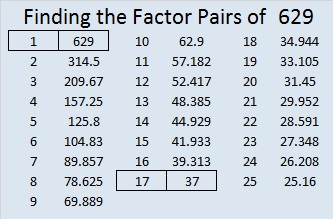# 629 and Level 1

629 is the sum of the 17 prime numbers from 7 to 71. Both of its prime factors, 17 and 37, are included in that list.

17 and 37 are both 10 numbers away from their average, 27. That means that 629 + 10² = 729 or 27².

25² + 2² = 629 and 23² + 10² = 629. Notice that 629 plus or minus 100 is a square number.

Both of 629’s prime factors have a remainder of one when divided by four so 629 is the hypotenuse of four Pythagorean triples, two of which are primitives.

• 100-621-629, a primitive that reminds me of another primitive, 20-21-29
• 204-595-629, three numbers whose greatest common factor is 17
• 296-555-629, three numbers whose greatest common factor is 37
• 429-460-629, a primitive whose shorter leg is exactly 200 less than its hypotenuse.Print the puzzles or type the solution on this excel file: 10 Factors 2015-09-28

—————————————————————————————————

• 629 is a composite number.
• Prime factorization: 629 = 17 x 37
• The exponents in the prime factorization are 1 and 1. Adding one to each and multiplying we get (1 + 1)(1 + 1) = 2 x 2 = 4. Therefore 629 has exactly 4 factors.
• Factors of 629: 1, 17, 37, 629
• Factor pairs: 629 = 1 x 629 or 17 x 37
• 629 has no square factors that allow its square root to be simplified. √629 ≈ 25.079872.—————————————————————————————————This site uses Akismet to reduce spam. Learn how your comment data is processed.